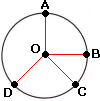## Definition Of Circle

A Circle is the locus of all points that are at an equal distance from a given point (on the plane) called the center.

The distance around a circle is called as its circumference.

### Example of Circle

In the figure shown, O is the center of the circle.
All points on the black ring, such as A, B, C, and D, are equidistant from O, the center. The length of the black ring is the circumference of the circle.A. 37.7 feet
B. 12 feet
C. 6 feet
D. 2 feet